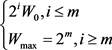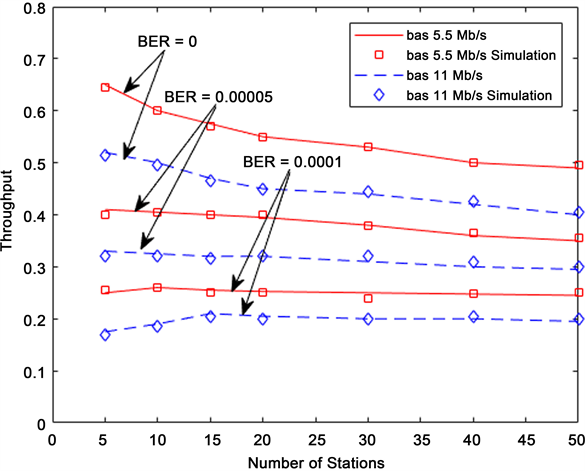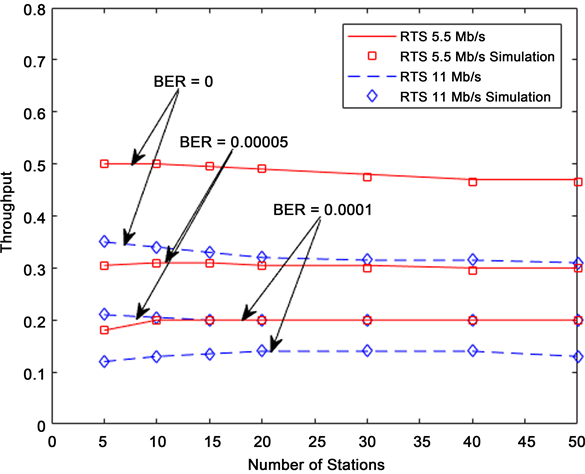# 基于指数退避算法的IEEE 802.11协议吞吐量分析Analysis of Throughput of IEEE 802.11 Protocol Based on Exponential Backoff Algorithm

• 全文下载: PDF(1166KB)    PP.64-69   DOI: 10.12677/HJWC.2018.82007
• 下载量: 450  浏览量: 1,688   国家自然科学基金支持

In the actual network communication environment, data packets cannot be efficiently transmitted due to channel interference, so it is necessary to improve the system performance impact under non-ideal channels. This paper takes the wireless LAN as the communication background, consi-dering the impact of non-ideal channels on WLANs such as signal fading and distortion, adds the freezing probability based on the previous literature exponential backoff algorithm to reduce the data collision in the channel and combine them. The two types of access methods are used to obtain the saturation throughput formula under the IEEE 802.11 protocol. Finally, simulation and analysis of the model are performed.

2. 改进后的模型分析(1)

$\left\{\begin{array}{l}P\left\{i,k|i,k+1\right\}=1-P\text{\hspace{0.17em}}\text{\hspace{0.17em}}\text{\hspace{0.17em}}\text{\hspace{0.17em}}\text{\hspace{0.17em}}\text{\hspace{0.17em}}\text{\hspace{0.17em}}\text{\hspace{0.17em}}\text{\hspace{0.17em}}\text{\hspace{0.17em}}\text{\hspace{0.17em}}\text{\hspace{0.17em}}\text{\hspace{0.17em}}\text{\hspace{0.17em}}\text{\hspace{0.17em}}\text{\hspace{0.17em}}k\in \left(0,{W}_{i}-\text{3}\right),\text{\hspace{0.17em}}i\in \left(0,m\right)\\ P\left\{0,k|i,0\right\}=\frac{\left(1-{P}_{r}\right)P}{{W}_{\text{0}}}\text{\hspace{0.17em}}\text{\hspace{0.17em}}\text{\hspace{0.17em}}\text{\hspace{0.17em}}\text{\hspace{0.17em}}\text{\hspace{0.17em}}\text{\hspace{0.17em}}\text{\hspace{0.17em}}\text{\hspace{0.17em}}\text{\hspace{0.17em}}\text{\hspace{0.17em}}\text{\hspace{0.17em}}\text{\hspace{0.17em}}k\in \left(0,{W}_{0}-\text{2}\right),\text{\hspace{0.17em}}i\in \left(0,m\right)\\ P\left\{i,k|i-1,0\right\}=\frac{{P}_{r}}{{W}_{\text{0}}}\text{\hspace{0.17em}}\text{\hspace{0.17em}}\text{\hspace{0.17em}}\text{\hspace{0.17em}}\text{\hspace{0.17em}}\text{\hspace{0.17em}}\text{\hspace{0.17em}}\text{\hspace{0.17em}}\text{\hspace{0.17em}}\text{\hspace{0.17em}}\text{\hspace{0.17em}}\text{\hspace{0.17em}}\text{\hspace{0.17em}}\text{\hspace{0.17em}}\text{\hspace{0.17em}}\text{\hspace{0.17em}}\text{\hspace{0.17em}}\text{\hspace{0.17em}}k\in \left(0,{W}_{0}-\text{2}\right),\text{\hspace{0.17em}}i\in \left(0,m\right)\\ P\left\{m,k|m,0\right\}=\frac{{P}_{r}}{{W}_{m}}\text{\hspace{0.17em}}\text{\hspace{0.17em}}\text{\hspace{0.17em}}\text{\hspace{0.17em}}\text{\hspace{0.17em}}\text{\hspace{0.17em}}\text{\hspace{0.17em}}\text{\hspace{0.17em}}\text{\hspace{0.17em}}\text{\hspace{0.17em}}\text{\hspace{0.17em}}\text{\hspace{0.17em}}\text{\hspace{0.17em}}\text{\hspace{0.17em}}\text{\hspace{0.17em}}\text{\hspace{0.17em}}\text{\hspace{0.17em}}\text{\hspace{0.17em}}\text{\hspace{0.17em}}k\in \left(0,{W}_{m}-\text{2}\right)\\ P\left\{-1,0|i,0\right\}=\left(1-P\right)\left(1-{P}_{r}\right)\text{\hspace{0.17em}}\text{\hspace{0.17em}}\text{\hspace{0.17em}}\text{\hspace{0.17em}}\text{\hspace{0.17em}}k\in \left(0,{W}_{i}-\text{2}\right)\end{array}$ (2)

3. 饱和吞吐量分析

${T}_{S}^{bas}=H+E\left[L\right]+\delta +{T}_{SIFS}+{T}_{ACK}+\delta +{T}_{DIFS}$ (3)

RTS/CTS接入方式：

${T}_{S}^{rts}={T}_{RTS}+\sigma +{T}_{SIFS}+{T}_{CTS}+\sigma +{T}_{SIFS}+H+E\left[L\right]+\delta +{T}_{SIFS}+{T}_{ACK}+\delta +{T}_{DIFS}$ (4)

 (5)

$P=1-{\left(1-\tau \right)}^{n}$ (6)

$\tau =\underset{i=-1}{\overset{m}{\sum }}{b}_{i,0}=\frac{\left(1-P{P}_{r}^{m+2}-\left(P+{P}_{r}\right){P}_{r}^{m+1}\right)\cdot \left(2-2P\right)\left(1-2{P}_{r}\right)}{2{\left(1-P\right)}^{2}\left(1-2{P}_{r}\right)\left(1-{P}_{r}\right)+\left(\left(1-{P}_{r}\right)P+{P}_{r}\right)\left(1-2{P}_{r}\right)\left({W}_{0}+1\right)+\left(\left(1-{P}_{r}\right)P+{P}_{r}\right)\left(1-{\left(2{P}_{r}\right)}^{m}\right){W}_{0}{P}_{r}}$ (7)

$\begin{array}{c}{P}_{r}^{bas}\\ {P}_{r}^{rts}\end{array}\right\}=\left\{\begin{array}{l}1-{\left(1-\tau \right)}^{n}{\left(1-BER\right)}^{H+E\left[L\right]+{T}_{ACK}}\\ 1-{\left(1-\tau \right)}^{n}{\left(1-BER\right)}^{{T}_{RTS}+{T}_{CTS}+H+E\left[L\right]+{T}_{ACK}}\end{array}$ (8)

${P}_{S}^{bas}=\frac{n\tau {\left(1-\tau \right)}^{n}{\left(1-BER\right)}^{H+E\left[L\right]+{T}_{ACK}}}{P}$ (9)

RTS/CTS访问方式成功传输概率：

 (10)

$S=\frac{E\left[数据成功传输的平均分组长度\right]}{E\left[数据成功传输的平均时延\right]}$ (11)

$\begin{array}{c}\begin{array}{c}{S}^{bas}\\ {S}^{rts}\end{array}\right\}=\frac{E\left[d\right]}{E\left[\sigma \right]}=\frac{{P}_{S}\cdot P\cdot E\left[L\right]}{\left(1-P\right)\sigma +{P}_{S}\cdot {T}_{S}\cdot P+\left(1-{P}_{S}\right)\cdot {T}_{C}\cdot P}\\ =\left\{\begin{array}{l}\frac{n\tau {\left(1-\tau \right)}^{n}{\left(1-BER\right)}^{{T}_{RTS}+{T}_{CTS}+H+E\left[L\right]+{T}_{ACK}}\cdot E\left[L\right]}{\left(1-P\right)\sigma +{P}_{S}\cdot {T}_{S}\cdot P+\left(1-{P}_{S}\right)\cdot {T}_{C}\cdot P}\\ \frac{n\tau {\left(1-\tau \right)}^{n}{\left(1-BER\right)}^{H+E\left[L\right]+{T}_{ACK}}\cdot E\left[L\right]}{\left(1-P\right)\sigma +{P}_{S}\cdot {T}_{S}\cdot P+\left(1-{P}_{S}\right)\cdot {T}_{C}\cdot P}\end{array}\end{array}$ (12)

4. 数值分析Figure 1. Saturation throughput graph for basic access methodFigure 2. Saturation throughput graph for RTS/CTS access method

5. 结束语

  Bianchi, G. (2006) Performance Analysis of the IEEE 802.11 Distributed Coordination Function. IEEE Press, New York, 18(3): 535-547.  Weng, C.E. and Chen, H.C. (2016) The Performance Evaluation of IEEE 802.11 DCF Using Markov Chain Model for Wireless LANs. Advanced Materials. Springer International Publishing, Germany, 144-149.  Tuysuz, M.F. and Mantar, H.A. (2015) Exploiting the Channel Using Uninterrupted Collision-Free MAC Adaptation over IEEE 802.11 WLANs. Wireless Commu-nications & Mobile Computing, 15, 889-909. https://doi.org/10.1002/wcm.2391  Hong, K., Kim, J.P., Kim, M.S., et al. (2016) Channel Measurement-Based Access Point Selection in IEEE 802.11 WLANs. Pervasive & Mobile Computing, 30, 58-70. https://doi.org/10.1016/j.pmcj.2015.10.018  Petruzzella, V., Vergari, R.I., Boffoli, D., et al. (2005) Saturation Throughput Analysis of IEEE 802.11 Wireless LANs for a Lossy Channel. IEEE Communications Letters, 9, 100-102. https://doi.org/10.1109/LCOMM.2005.02011  Panthum, T., Sittichivapark, S. and Sartthong, J. (2016) Performance Anal-ysis of EIED Backoff Algorithm of the IEEE 802.11 MAC under Fading Channel Errors. International Joint Conference on Computer Science and Software Engineering, IEEE, 1-6.